# Testing equivalence with TOST in Excel tutorial

2017-10-20

This tutorial will help you run and interpret an equivalence test (TOST) to validate the difference between two means, in Excel using the XLSTAT software.
Not sure this is the statistical test you are looking for? Check out this guide.

## What is the TOST equivalence test?

The XLSTAT equivalence test allows to test if two products are equivalent. TOST means two-one-sided-test.

This type of test is used primarily to validate bioequivalence. When we want to show the equivalence of two drugs, classical hypothesis testing does not apply, we will use equivalence testing which will validate the equivalence between the two drugs.

XLSTAT offers two equivalent methods to test equivalence using the TOST test.

• Using the 100 * (1-2 * alpha)% confidence interval around the mean. By comparing this interval to the user-defined interval of equivalence, we can conclude the equivalence or non equivalence. Thus, if the confidence interval is within the interval defined by the user, we conclude the equivalence between the two samples. If one of the bounds of the confidence interval is outside the interval defined by the user, then the two samples are not equivalent.
• Using two one-sided tests, one on the right and one on the left. We apply a right one-sided t-test on the lower bound of the interval defined by the user and a left one-sided t-test on the upper bound of the interval defined by the user. We obtain p-values for both tests. We take the greatest of these p-values as p-value of the equivalence test.

## Dataset to run a TOST equivalence test on two independent samples

An Excel sheet containing both the data and the results for use in this tutorial can be downloaded by clicking here.

The data represent the time that a customer will spend in a restaurant depending on the type of wine that is offered (red or white). The restaurant owner want to have his customer stay longer so that they spend more money in his establishment. There are 42 customers that have been studied.

We want to know if the fact that a customer chooses red or white wine is equivalent regarding the time spent in the restaurant. The difference should not exceed 10 minutes, we choose to have a TOST interval of [-10; 10].

## Setting up a TOST equivalence test

After opening XLSTAT, select the XLSTAT / Parametric tests / TOST (Equivalence test) command, or click on the corresponding button of the Parametric tests toolbar (see below).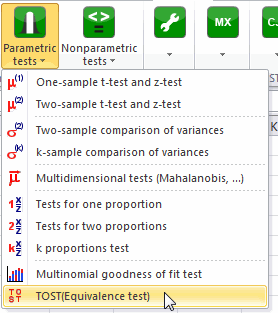Once you've clicked on the button, the dialog box appears.

We select one column in each box.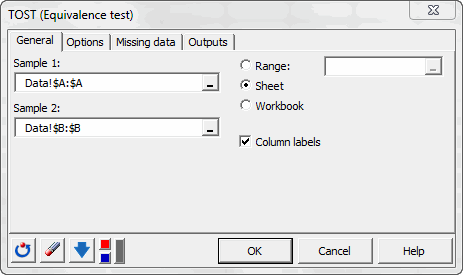In the Options tab, we want to test a difference of 0 and the bounds of the equivalence test are -10 and 10.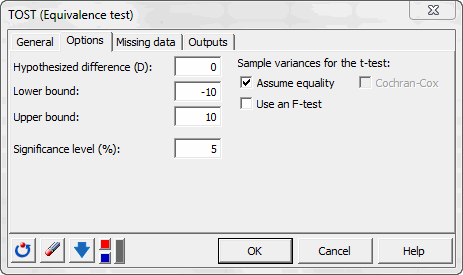The computations begin once you have clicked on OK. The results will then be displayed in a new sheet.

## Interpreting the results of an equivalence (TOST) test on two independent samples

The first results displayed are the statistics for the various samples. Next, the equivalence test is displayed. There are two equivalent results to interpret the TOST.

The first table displays the 90% confidence interval of the mean and compares it to the user defined TOST interval.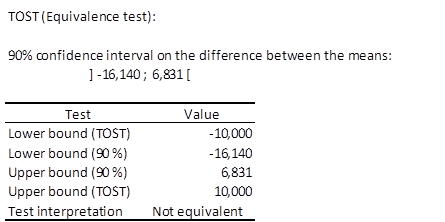In order to have equivalence between red and white wine, the 90% confidence interval has to be included in the TOST interval. We can see that -16,140<-10, so the equivalence is not accepted.

The second table is equivalent to the first one, but using one-sided t-test with associated p-values.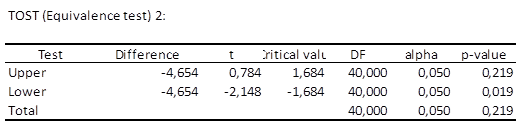We see that with a total p-value of 0.219, the equivalence is rejected.

We can conclude that offering white or red wine is not equivalent regarding the time spent in the restaurant by the customers.

#### Contact our technical support team: support@xlstat.com

https://cdn.desk.com/
false
desk